## Abstract

In this paper, a tower solar collector-aided coal-fired power generation (TSCACPG) system is proposed and studied in order to save the fossil energy and protect the environment. The integration scheme of tower solar collector and conventional coal-fired power plant is proposed. Based on the simulation platform TRNSYS, the TSCACPG system model is established and the dynamic performance of the TSCACPG system with the operating mode of coal saving is studied. The TSCACPG system performances of 1 day and 1 year are all discussed by using the DNI data of typical year in Chinese typical city of Dunhuang. Then, the sensitivity analysis of the TSCACPG system is carried out by changing the heliostat field scale (the size of the molten salt tower also changes accordingly). The annual performance of the TSCACPG system is also acquired. In consideration of the economic costs, the heliostat field area with the maximum annual solar-to-electric efficiency is selected as the optimal value. The results show that, for the case studied, the optimal heliostat field area is 101,400 m2, and the maximum annual solar-to-electric efficiency is 16.74%. And under the optimal situation, the standard coal consumption rate of the original coal-fired power plant is reduced from 301.5 g/kWh to 294.5 g/kWh.

## Introduction

Over a long period, the fossil fuel as the primary energy has played a key role in the human survival and social development, but at the same time, it is accompanied by the serious environment pollution problem and great energy consumptions [1, 2]. In order to realize the sustainable development of the living environment, new energy resources should be explored and the clean renewable energy should be utilized on a larger scale in order to gradually reduce the destruction of the environment and the dependence on the fossil energy . The complementary utilization of the renewable energy and the fossil energy has gradually become an important development trend of the future energy system [4-7]; especially in China, about 70% of the electricity comes from coal-fired power plants. Currently, restricted by the resource shortage and environment pollution problems, many medium and small thermal power plants that consume a large amount of coal will face the fate of shutting down. The utilization of the solar energy with its unique advantage has been paid more and more attentions. The solar energy is inexhaustible energy without greenhouse gases (mainly CO2, NOX) or toxic gases (SO2 and particulate) emissions. As a kind of special renewable energy, the solar energy is not only abundant but also cheap .

Presently, the solar thermal power generation has been widely applied and developed at home and abroad, such as the power generation of solar parabolic trough and solar energy tower . But for the independent solar thermal power generation system, both the high initial investment and lower thermal performance are major obstacles to its development . However, the solar energy-aided power generation system can integrate the solar energy into a fossil fuel (coal or gas)-fired power plant at a relatively low cost . For instance, the United States Colorado project that started in July 2010 is the first solar-aided coal-fired power plant with the grid-connected power generation mode. The heat capacity is 4 MW (total capacity of power station is 44 MW), and the collector area is 25,899 m2. The power plant efficiency is improved by 3–5%, and 2000 tons per year of greenhouse gas emissions is reduced. In addition, the huge power generation project of Kogan Creek Solar Boost, combination of 750-MW coal-fired power station and 44-MW solar heat collection system, is located in Queensland, Australia, adopting the CLFR type (linear Fresnel) technology. The collector area is about 300,000 m2, and the annual power generation capacity is 44 GWh. The reduced carbon dioxide emission is 35,600 tons per year, and the investment cost is \$105 million.

In recent years, many researches have been carried out on the integration of the solar thermal energy with the traditional fossil fuel-fired power system. A typical manner to employ the solar-aided coal-fired power generation technique is to heat the boiler feedwater using the solar thermal energy instead of the regenerative extraction steam in the coal-fired power unit. In the studies of Y. Yang team and H. Jin team, the solar heat with about 300°C medium-or-low temperature is added into the regenerative system of the conventional power plant by using the trough solar collectors [12, 13]. Hou et al. and Peng et al. also studied the solar-aided coal-fired power generation system on the basis of the second law of thermodynamics [14, 15]. Gupta and Kaushik concluded that using the solar energy heating the boiler feedwater of a thermal power plant is more efficient than using the same solar energy in a stand-alone solar thermal power plant . Eck and Zarza proved the feasibility of the direct steam generation process in a horizontal parabolic trough concentrator . Popov Dimityr studied the three replacements of low-pressure heaters, high-pressure heaters, high-pressure heaters and economizer by using the solar energy, respectively . Jian et al.  analyzed the performance of a trough solar energy collector-aided 330-MW plant with part of extraction steam in high-pressure (HP) heaters replaced in the fuel-saving operation mode. Larrain et al.  established the 100-MW fossil energy-aided feedwater heating of solar thermal power plant, and using the climatic parameters of Chile desert to find the best efficiency in the hybrid system with the minimum amount of fossil energy. Zhao et al.  analyzed the process of energy transfer of the solar-aided coal-fired power generation system, and the reason why the thermodynamic performance of the solar-aided system is better than that of the original unit is revealed.

Although many researches on the integration of the parabolic trough solar energy collector system with the traditional coal-fired power plant, there is few study on the tower solar collector-aided coal-fired power generation system. And in the previous researches, the impact of DNI is usually neglected. In this paper, the high-temperature tower solar energy collector is integrated into the superheating and reheating system of the boiler, and the impact of variation in DNI data is considered. The conventional coal-fired power plant system integrated with the tower solar energy collector with the molten salt as the working medium is discussed. In consideration of the variation in DNI and heliostat field area, the dynamic performance of the overall system is analyzed in order to seek the best annual performance at the design point. In addition, the standard coal consumption rate of the overall system is also discussed in this paper.

The novelty of this paper lies in the following several aspects:

1. The coal-fired power plant integrated with tower solar collector with the operating mode of coal saving is proposed and deeply investigated.
2. The solar radiation intensity is adequately considered in the annual performance analysis.
3. On the basis of the annual performance, the heliostat field area is optimized and discussed.

## System Descriptions

### Coal-fired power generation system (base system)

A 660-MW supercritical coal-fired power unit is selected as the base system. Its feedwater regenerative part includes three high-pressure feedwater heaters, one deaerator, and four low-pressure feedwater heaters. The simplified scheme of the coal-fired power generation system and its main parameters is shown in Figure 1 and Table 1, respectively.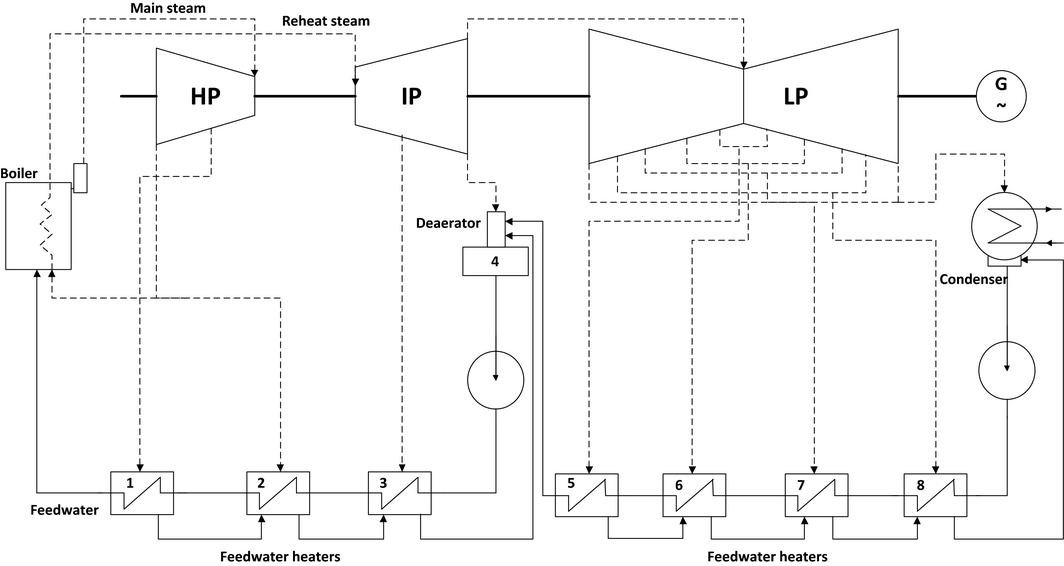Figure 1. Schematic diagram of the coal-fired power generation system.

Table 1. Main parameters of coal-fired power plant at design point (rated load)
Item Unit Value
Power MW 660
Main steam pressure MPa 24.2
Main steam temperature °C 566
Reheat steam temperature °C 566
Boiler feedwater temperature °C 275.3
Mass flow rate of the boiler feedwater t/h 2150.6
Turbine exhausted steam pressure kPa 5.3
Turbine exhausted steam enthalpy kJ/kg 2351.5

### The integration of tower solar collector system with the boiler system of the base system

In this paper, the tower solar collector system uses the molten salt as the working medium to absorb the solar energy and release the heat energy to the steam from the base coal-fired power generation system. And two solar energy heaters are adopted. Figure 2 shows the local schematic diagram of the integration of the tower solar collector system with the boiler system of the base system.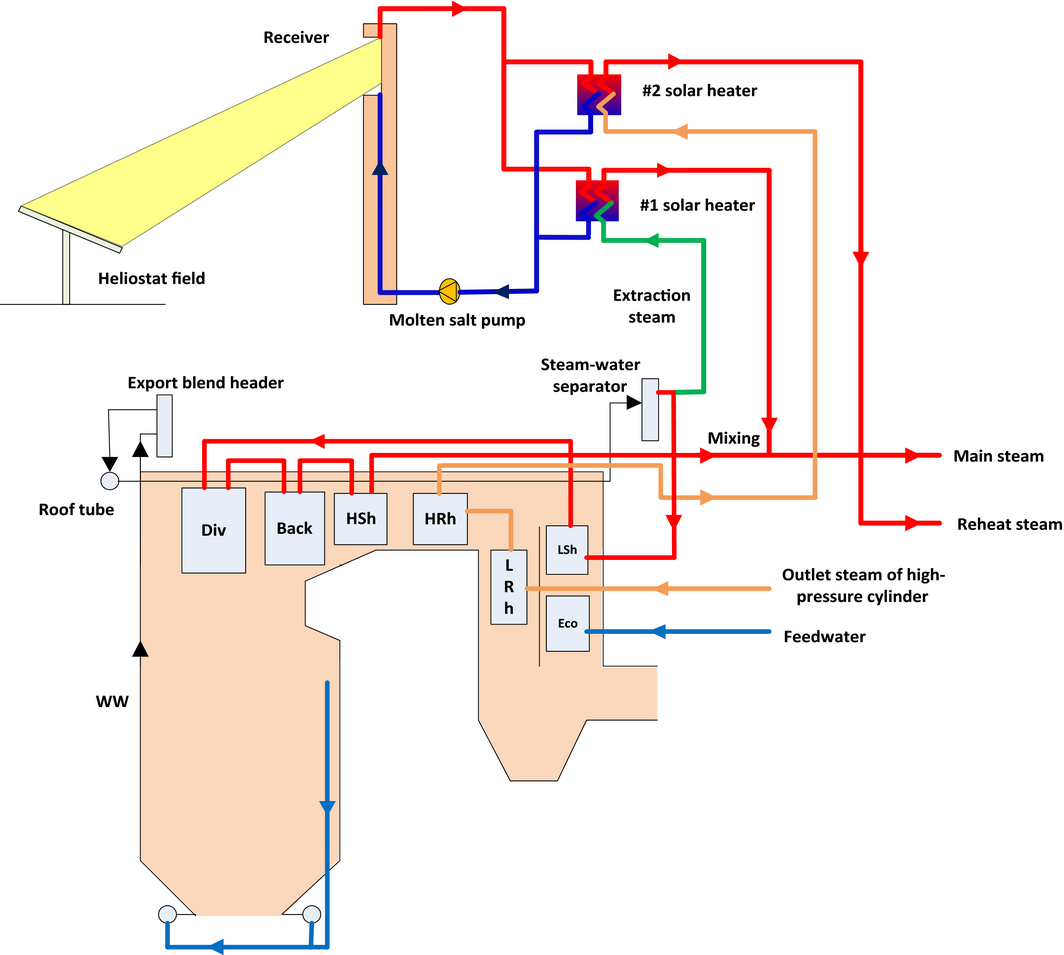Figure 2. Local schematic diagram of integration of the solar tower system with the boiler system of the base system.

Part of the extraction steam from the boiler steam-water separator is heated by the #1 solar heater and then mixes with the outlet steam of the boiler high-temperature superheater with the reduced steam temperature because of the operation mode of coal saving in order to maintain the temperature of the main steam (high-pressure cylinder inlet steam) at the original level (566°C). The #2 solar heater heats the outlet steam of high-temperature reheater with the reduced steam temperature because of the operation mode of coal saving in order to maintain the reheat steam temperature at the original level (566°C).

### TSCACPG system

In this paper, a tower solar collector-aided coal-fired power generation (TSCACPG) system is proposed and studied with the operating mode of coal saving (the boiler feedwater flow rate is constant, and the coal consumption rate is reduced with the introduction of solar energy). Figure 3 shows the schematic diagram of the TSCACPG system.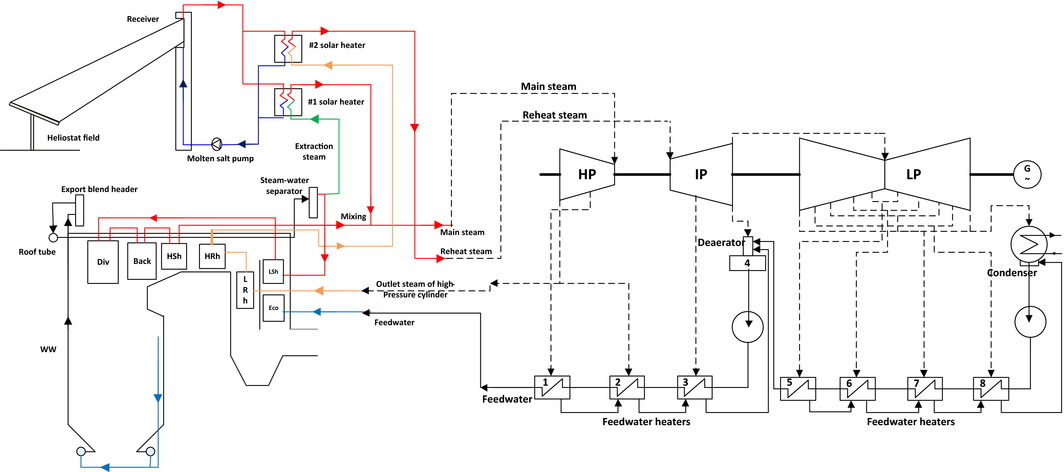Figure 3. Schematic diagram of the TSCACPG system.

The main advantages of the TSCACPG system lie in the following three aspects.

1. Firstly, compared with the reheat steam temperature adjustment mode by using the flue gas damper, the TSCACPG system avoids the disadvantages of a large time lag and the limited adjustment range.

As we know, the reheat steam temperature adjustment method with the flue gas damper has the advantages of simple structure and convenient operation, which is used by many large-scale power plant boilers. But both the opening degree of the flue gas damper and the change of steam temperature are nonlinear, and the effective range is narrow. Generally, the flue gas damper has a good performance in the 40~60% opening degree. But, if the opening degree is below 25% or above 75%, the flue gas damper will be useless. This kind of the reheat steam temperature adjustment method will be ineffective and cannot guarantee the rated reheat steam temperature. As in the actual operation, there will be some fluctuations in the coal and the load. After that, both the composition of flue gas and the opening degree will be changed. Coupled with the instability of solar energy, a higher requirement for the sensitivity and the damper adjustment speed is put forward. In this paper, the #2 solar heater is used to adjust the reheat steam temperature; at the same time, the flue gas damper is only used as an auxiliary temperature adjustment method. So it avoids the above-mentioned problem.

2. Secondly, compared with heating the feedwater or condensation water mode by using the solar energy, because of without the change in the regenerative extraction steam flow, the TSCACPG system will not affect the changes in steam flow, pressure, temperature and the output power of the steam turbine. And it is not necessary to calculate and evaluate the steam turbine performance at all levels once again to ensure the safe operation of the unit.

3. Thirdly, as the supercritical once-through boiler has no steam drum, the heat storage capacity is small. Therefore, in the same degree of disturbance, the steam temperature changes more abruptly. And the TSCACPG system can better control the temperatures of both the main steam and reheat steam.

## Simulation of the System

In this paper, the TSCACPG system is simulated with the software platform TRNSYS that has been a perfect transient simulation software with the extended function developed by the University of Wisconsin. And Figure 4 is the TSCACPG simulation system built by the TRNSYS.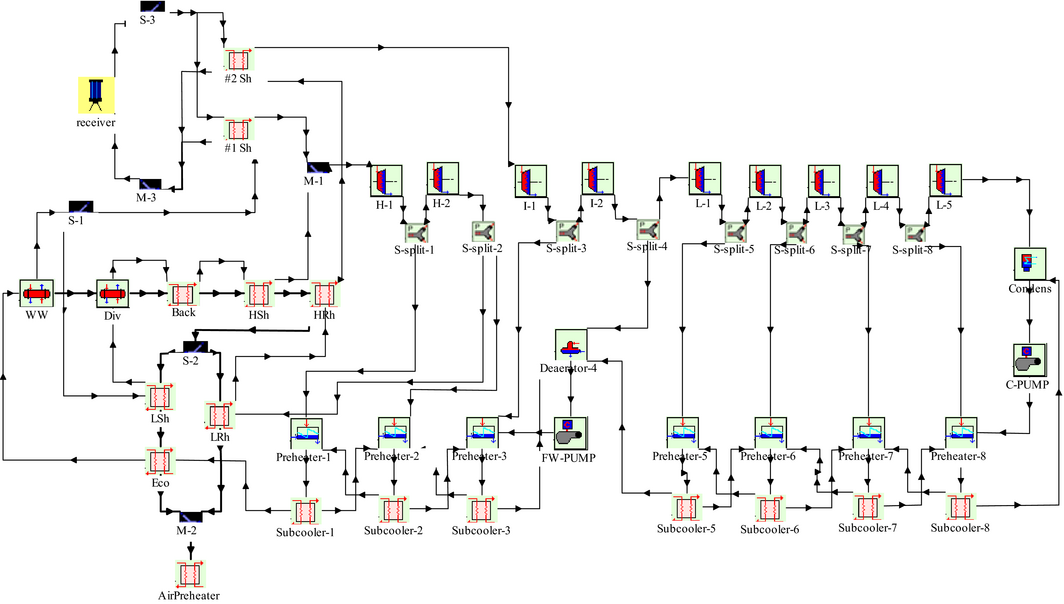Figure 4. The TSCACPG simulation system built by TRNSYS.

### Heliostat field

The heliostat field model requires a user-supplied field efficiency matrix to evaluate the field efficiency, ηfield, as a function of the date and time. The field efficiency matrix will be read in and interpolated by the TRNSYS routine data. It comes from the actual operating parameters of a solar power station. Its specific values are shown in Table 2. And its three-dimensional graph is shown in Figure 5. The power to the receiver is calculated by the following equation:

 ${\dot {Q}}_{rec}=A_{field}\cdot {\rho }_{field}\cdot I\cdot {\eta }_{field}\cdot \Gamma .$
(1)

Table 2. The field efficiency 7 × 9 matrix including 7 zenith angles and 9 azimuth angles
−120 −90 −60 −15 0 15 60 90 120
0 0.1710 0.1430 0.1523 0.1782 0.3187 0.3658 0.1803 0.1523 0.1482
25 0.5513 0.6363 0.6839 0.7140 0.7280 0.7264 0.6373 0.5710 0.4819
45 0.6352 0.6974 0.7409 0.7751 0.7808 0.7793 0.7130 0.6528 0.5855
65 0.6839 0.7171 0.7440 0.7658 0.7705 0.7699 0.7316 0.6964 0.6611
75 0.7016 0.7212 0.7378 0.7513 0.7554 0.7565 0.7337 0.7119 0.6912
85 0.7140 0.7202 0.7264 0.7306 0.7342 0.7368 0.7285 0.7223 0.7150
90 0.7223 0.7223 0.7223 0.7223 0.7223 0.7223 0.7223 0.7223 0.7223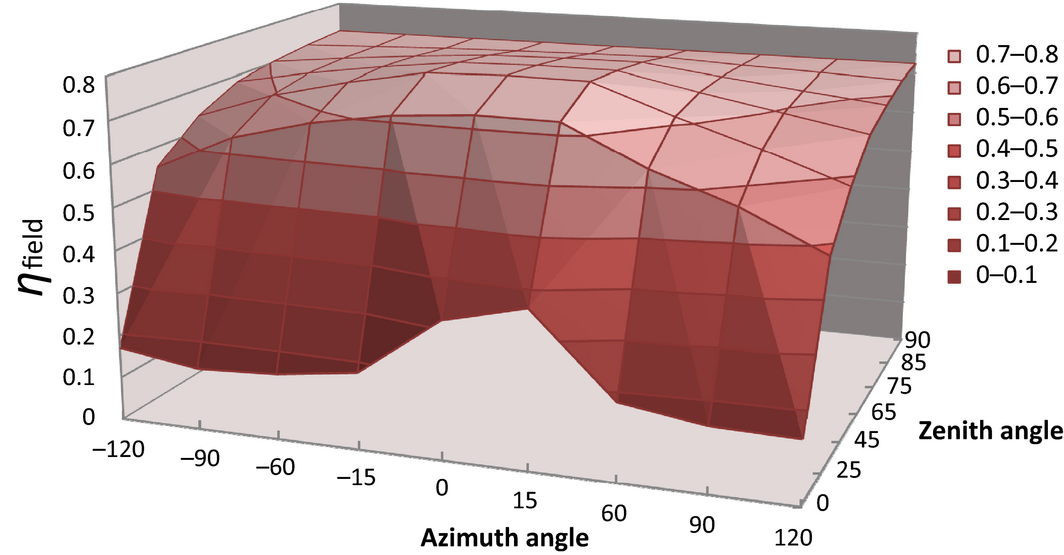Figure 5. The three-dimensional graph of the field efficiency matrix.

The model also considers the parasitic electrical energy consumption for tracking, start-up and shutdown. The shutdown is performed automatically at the high wind speed .

The receiver is a photo-thermal conversion device in the solar power tower system. The molten salt, which consists of LiCl and KCl (the component ratio of LiCl and KCl is 0.595:0.405, and the working temperature ranges from 355°C to 1400°C), is used as the working medium of tower solar collector. The outlet temperature of the molten salt receiver is designed to a constant value of 620°C.

The receiver outlet parameters are calculated depending on the inlet condition of the molten salt and the radiation input. And the receiver body and piping heat losses can be calculated .

The model of the receiver in the paper is described as follows:

 ${\overline {T}}_{abs}={\frac {t_{in}+t_{out}}{2}}+273.15$
(2)
 ${\dot {Q}}_{{loss}_{-}rad}=A_{p}\cdot eps_{abs}\cdot 3600\cdot \sigma \cdot {\left({\overline {T}}_{abs}\right)}^{4}$
(3)
 ${\dot {Q}}_{abs}=eta_{opt}\cdot {\dot {Q}}_{rec}-{\dot {Q}}_{{loss}_{-}rad}$
(4)
 ${\dot {Q}}_{{loss}_{-}pipe}=A_{pipe}\cdot \left(e,p,s_{pipe},\cdot ,3600,\cdot ,\sigma ,\cdot ,{\left(t_{out}+273.15\right)}^{4},+\right.$$\left.,k_{pipe},\cdot ,\left(t_{out}-t_{amb}\right)\right)$
(5)
 ${\dot {Q}}_{{loss}_{-}cool}=f_{{cooling}_{-}loss}\cdot {\dot {Q}}_{abs}$
(6)
 ${\dot {Q}}_{net}={\dot {Q}}_{abs}-{\dot {Q}}_{{loss}_{-}pipe}-$${\dot {Q}}_{{loss}_{-}cool}$
(7)
 ${\dot {Q}}_{th}=c_{salt}\cdot {\dot {m}}_{salt}\cdot \left(t_{out}-\right.$$\left.t_{in}\right)$
(8)
 ${\eta }_{rec}={\frac {{\dot {Q}}_{net}}{{\dot {Q}}_{rec}}}$
(9)

### #1 solar heater

The #1 solar heater heats the extraction steam from the boiler steam-water separator. The model adopted for the #1 solar heater in the paper is described as follows:

 ${\dot {m}}_{ms1}\cdot \left(h_{i,ms1}-h_{o,ms1}\right)=$${\dot {m}}_{es}\cdot \left(h_{0,es}-h_{1,es}\right)$
(10)

### #2 solar heater

The #2 solar heater heats the outlet steam of high-temperature reheater. The model adopted for the #2 solar heater in the paper is described as follows:

 ${\dot {m}}_{ms2}\cdot \left(h_{i,ms2}-h_{o,ms2}\right)=$${\dot {m}}_{rs}\cdot \left(h_{o,rs}-h_{i,rs}\right)$
(11)

### System performance evaluation

To evaluate the benefits or the efficiency of the solar heat utilization in the TSCACPG, the solar-to-electricity efficiency (ηse) is defined as:

 ${\eta }_{se}={\frac {3.6\cdot P_{S}}{{\dot {Q}}_{S}}}={\frac {3.6\cdot \left(P_{Z},-,{\frac {{\dot {Q}}_{b}}{3.6}},\cdot ,{\eta }_{ref}\right)}{{\dot {Q}}_{S}}}=$${\frac {3.6\cdot P_{Z}-{\dot {Q}}_{b}\cdot {\eta }_{ref}}{{\dot {Q}}_{S}}}$
(12)
 ${\dot {Q}}_{S}=A_{field}\cdot {\rho }_{field}\cdot I$
(13)

The standard coal consumption rate of the power plant is the boiler coal consumption for per kWh electricity output (g/kWh), defined as:

 $b={\frac {1000\quad m_{sc}}{E}}$
(14)

## Case Study

In this case, a tower solar energy collector-aided a 660-MW supercritical coal-fired power unit in Dunhuang, China (40°N and 94°E), is described and exemplified. Dunhuang is a city with rich solar resources. Figure 6 shows the DNI data of a typical year in Dunhuang.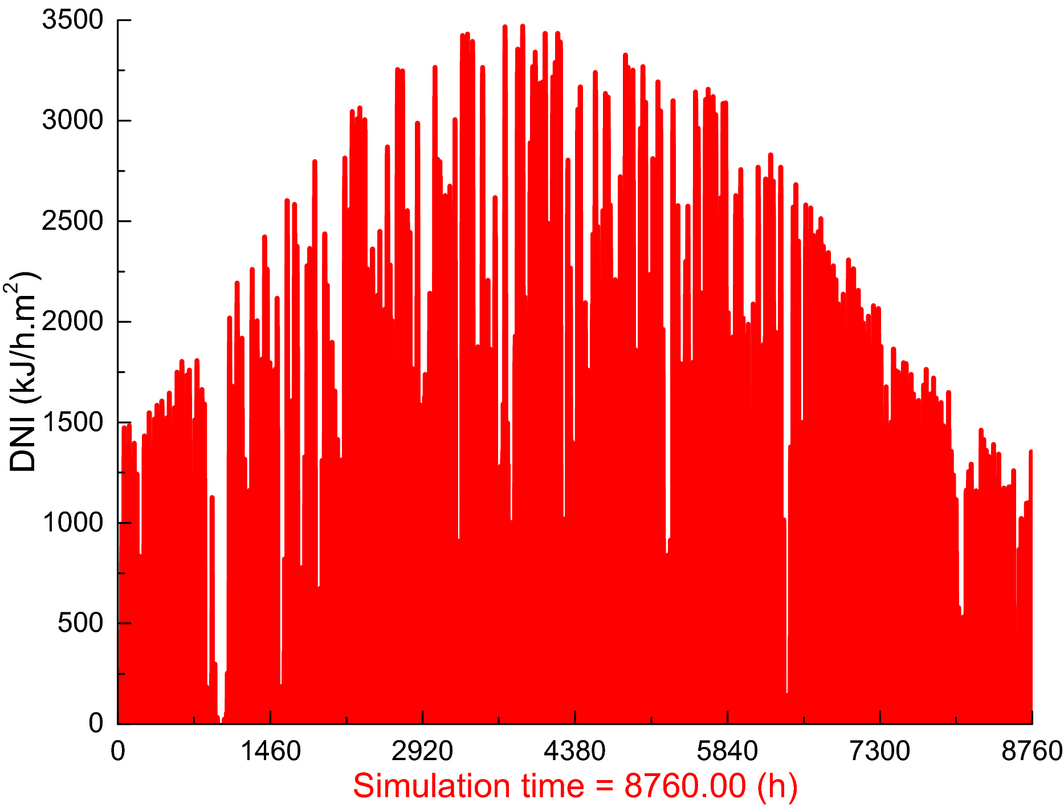Figure 6. DNI data of typical year in Dunhuang (kJ/h.m2).

### Size selection of tower solar energy collector system

It is necessary to determine an appropriate size of the heliostat field (molten salt tower size also changed accordingly) in order to match the 660-MW supercritical coal-fired power unit. The direct normal irradiance (DNI) of the sun is the prerequisite for the design of the heliostat field. This paper uses the weather database of TRNSYS and selects the DNI value 2499 kJ/h.m2 (equal to 694 W/m2) as the heliostat field design point. It is at 13:00 on 21 June, that is, the summer solstice day (4104–4128 h) in Dunhuang of the typical year data, as shown in Figure 7. According to the characteristics of the scheme, it is preliminarily determined that the heliostat surface area of 120,000 m2 and the molten salt mass flow rate of 1.80 × 106 kg/h are set as the reference for the performance analysis. In the later section of heliostat area optimization, the heliostat surface area will be further optimized.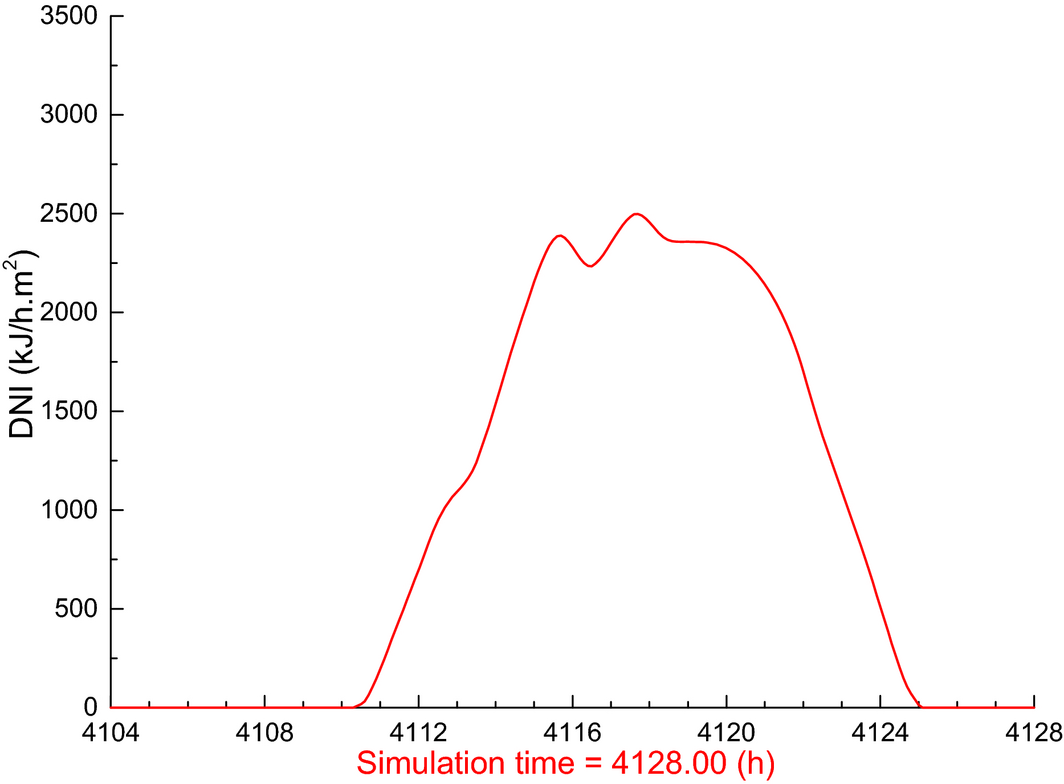Figure 7. DNI data of the summer solstice in Dunhuang (kJ/h.m2).

### Selection of the boiler extraction steam fraction

As we know, if the flue gas temperature of the power plant is too low, it will cause the serious low-temperature corrosion. And the temperature fluctuation of boiler heat exchanger will be great if the high-extraction steam fraction is selected. In this paper, the flue gas temperature being higher than 120°C and the temperature fluctuation of high-temperature superheater (HSh) being lower than 5°C are used as the constraint conditions to select the largest extraction steam fraction with the change in the heliostat field area. Under the heliostat surface design area of 120,000 m2, the extraction steam fraction of the boiler is set at 11%. And Table 3 shows the changes in temperature with the extraction steam fraction of 11%.

Table 3. The temperature changes of main parameters with 11% extraction steam
Temperature (°C) Original system After solar-aided Variation
Inlet steam of HP 566 566 +0
Inlet steam of IP 566 566 +0
Outlet steam of HSh 566 563.7 −4.25
Outlet steam of HRh 566 550.3 −15.7
Outlet steam of LRh 438.1 426.0 −12.1
Feedwater 275.3 275.3 +0
Flue gas 142.8 128.3 −14.5

### Performance analysis

The performance of the TSCACPG system is analyzed at the full load condition with the coal-saving operating mode (the boiler feedwater mass flow rate is constant and the coal consumption rate is reduced).

The logic flowchart for TRNSYS to carry out the analysis is shown in Figure 8. The loop algorithm is the iterative method. When the error is <0.01°C, the iteration terminates.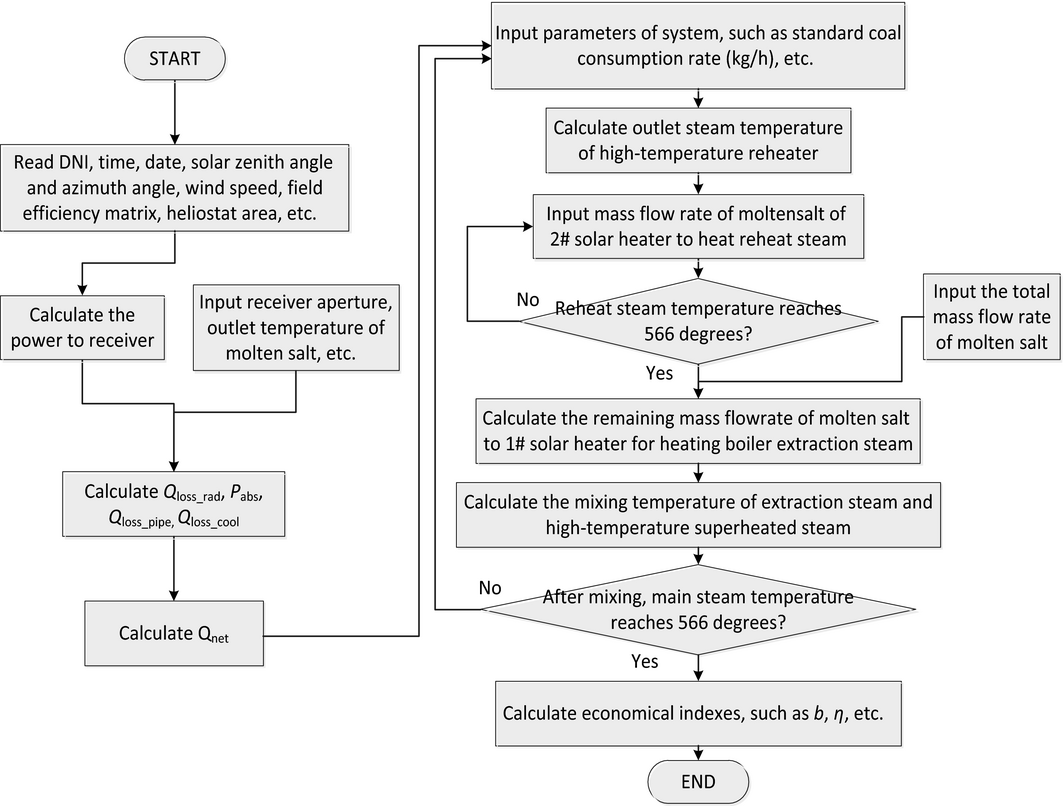Figure 8. Logic flowchart for the integrated system performance analysis.

Figure 9 shows the thermal load variations in the boiler heat exchangers. After adopting the coal-saving operating mode, the thermal load of each boiler heat exchanger declines from 1.2% of Eco to 15.1% of LSh. And the total decline is 40.8 MW with the reduction rate of 3.2%.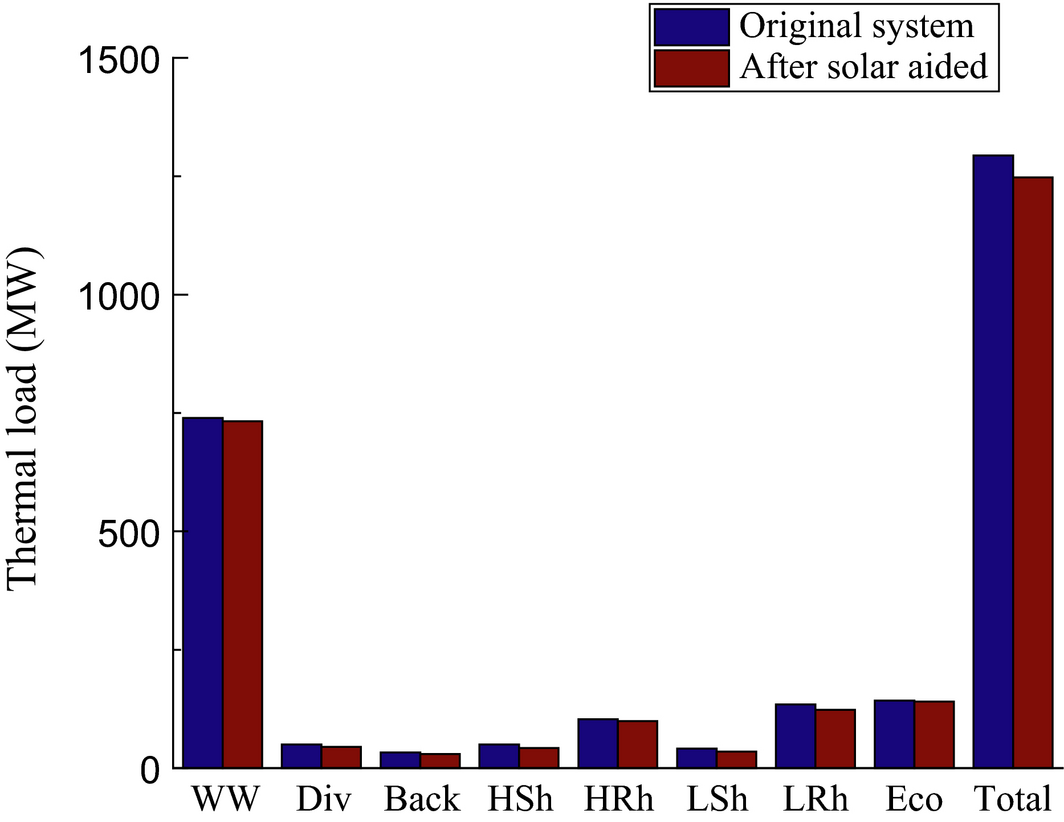Figure 9. The variations in thermal load of boiler heat exchangers.

Figure 10 shows the molten salt flow distributions of #1 and #2 solar heater. In Figure 10, L1 is the mass flow rate of molten salt for #1 solar heater (${\textstyle {\dot {m}}_{ms1}}$); L2 is the mass flow rate of molten salt for #2 solar heater (${\textstyle {\dot {m}}_{ms2}}$); LZ is the total mass flow rate of molten salt (${\textstyle {\dot {m}}_{salt}}$). This figure also reveals the scheme of start-up and shutdown. When the DNI value is greater than 1405 kJ/h.m2 (equal to 390 W/m2), the solar power generation system will be put into the conventional coal-fired power system; when the DNI value is less than 1405 kJ/h.m2, the solar power generation system will be removed. It should be noted that the outlet temperature and outlet flow rate of the molten salt of receiver remain unchanged during the stable operation. This means a portion of solar energy is not used when the DNI value exceeds the value of 2030 kJ/h.m2. The main reason is for the consideration of the stable and safe operation of the overall power plant system. In this paper, for the economic reason, the TSCACPG system without the energy storage unit is established. In addition, on the basis of the data from the literature , the additional electrical consumption (losses) to prevent the crystallization of the heat transfer medium in our study is assumed as 1% of the solar power output, which is considered in the simulation.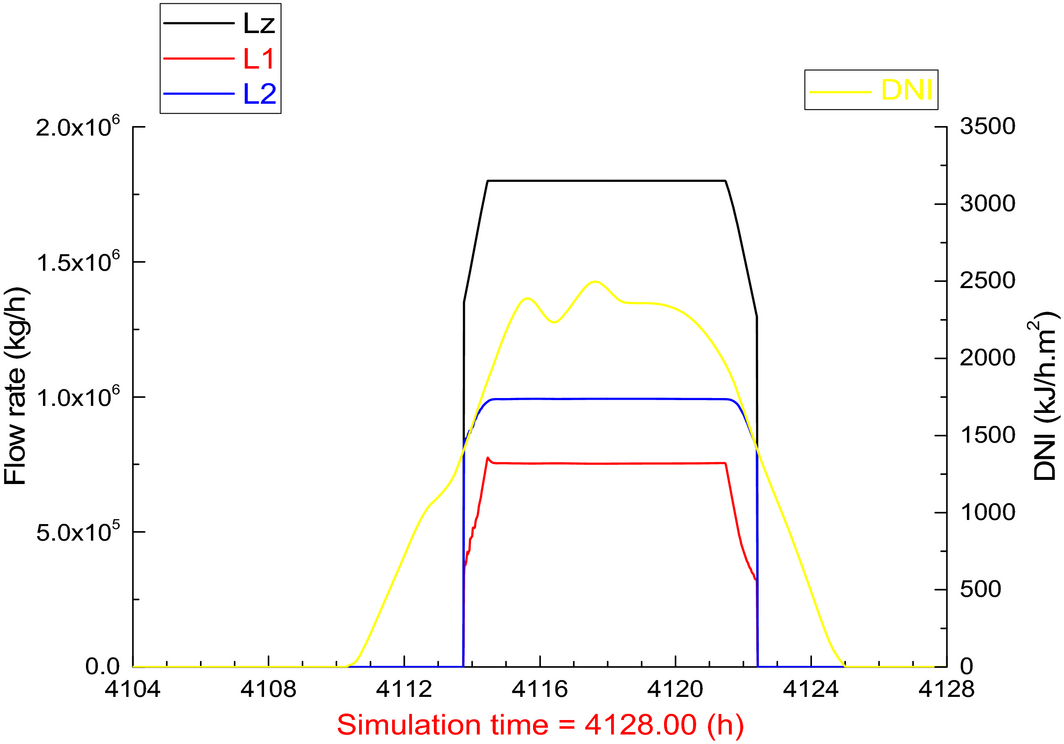Figure 10. Molten salt flow distributions of #1 and #2 solar heater.

Figure 11 shows both the efficiency and power of the tower solar energy system. In Figure 11, the field power is the heliostat field power (${\textstyle {\dot {Q}}_{rec}}$); the rec_power is the molten salt tower power (${\textstyle {\dot {Q}}_{net}}$); ms_abs_power is the molten salt tower thermal power (molten salt absorbed power) (${\textstyle {\dot {Q}}_{th}}$); the field_efficiency is the heliostat field efficiency (ηfield); the rec_efficiency is the molten salt tower efficiency (ηrec). The molten salt tower power is the result of DNI and the heliostat field efficiency factors. And the efficiency of molten salt tower is close to 90%. The thermal power of the solar power tower is 1.49 × 108 kJ/h (equal to 41.39 MW). The solar power generation system runs for 8.7 h on the summer solstice day.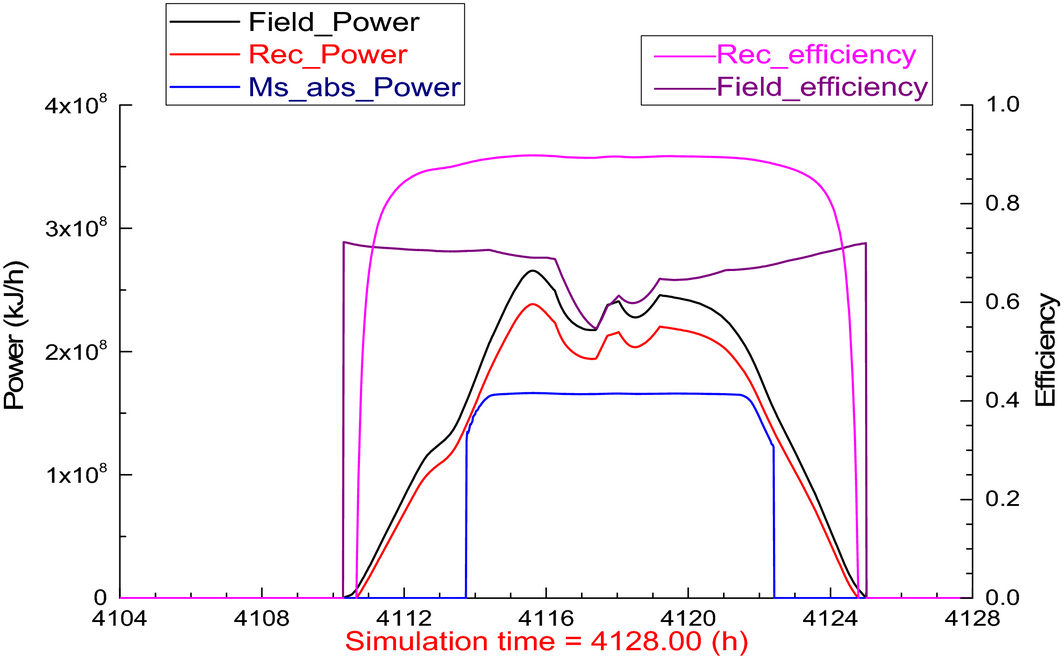Figure 11. Efficiency and power of the solar power generation system.

The simulation results are listed in Table 4. From Table 4, it can be seen that the net plant efficiency is increased by 1.07% and the standard coal consumption declines to 7.7 g/kWh correspondingly due to the aid of solar energy.

Table 4. Performance of the TSCACPG system at the design point
Items Unit Value
Power output of original unit MW 660
Power output of solar-aided unit (TSCACPG system) MW 660
Boiler feedwater mass flow rate t/h 2150.6
Inlet temperature of molten salt for #1 solar heater °C 620
Outlet temperature of molten salt for #1 solar heater °C 516.9
Inlet temperature of molten salt for #2 solar heater °C 620
Outlet temperature of molten salt for #2 solar heater °C 572.8
Outlet temperature of boiler extraction steam for #1 solar heater °C 600.4
Outlet temperature of reheat steam for #2 solar heater °C 566
Heliostat field area m2 121,700
Thermal power of solar power tower MW 41.39
Solar-to-electric efficiency  % 22.92
Standard coal consumption of original unit g/kWh 301.5
Standard coal consumption of solar-aided plant g/kWh 293.8
Standard coal consumption decline g/kWh 7.7
Net efficiency of original plant  % 40.80
Net efficiency of solar-aided plant (TSCACPG system)  % 41.87

In addition to the performance of the summer solstice day, the annual performance is also very important for the TSCACPG system. Therefore, the annual performance of the TSCACPG has been calculated using the DNI data of typical year in Dunhuang. Figure 12 shows the monthly utilization hours of the solar energy, and the annual performances are shown in Table 5. It can be seen that the annual utilization hours of solar energy reaches 2047 h and the annual solar-to-electric efficiency reaches to 15.14%.Figure 12. Monthly utilization hours of the solar energy.

Table 5. The annual performance of the TSCACPG system
Item Unit Value
Annual utilization hours of solar energy h 2047
Annual power generation of solar energy kWh 3.72 × 107
Annual solar-to-electric efficiency  % 15.14
Annual standard coal saved t 10,414

### Heliostat area optimization

The DNI of each day in a year is different, so the heliostat surface area mentioned above is not the optimal value which is designed on the summer solstice day. In order to get the optimal value, it is necessary to analyze the annual performance (such as the annual power generation, annual solar-to-electric efficiency) with different heliostat surface areas. In consideration of the economic cost, the area with the maximum annual solar-to-electric efficiency is selected as the optimal value. Figure 13 shows the variation in the boiler extraction steam fraction under different heliostat surface areas. Then, the correlation between the annual performance and the heliostat surface area is analyzed. And the analysis results are shown in Figures 14-16.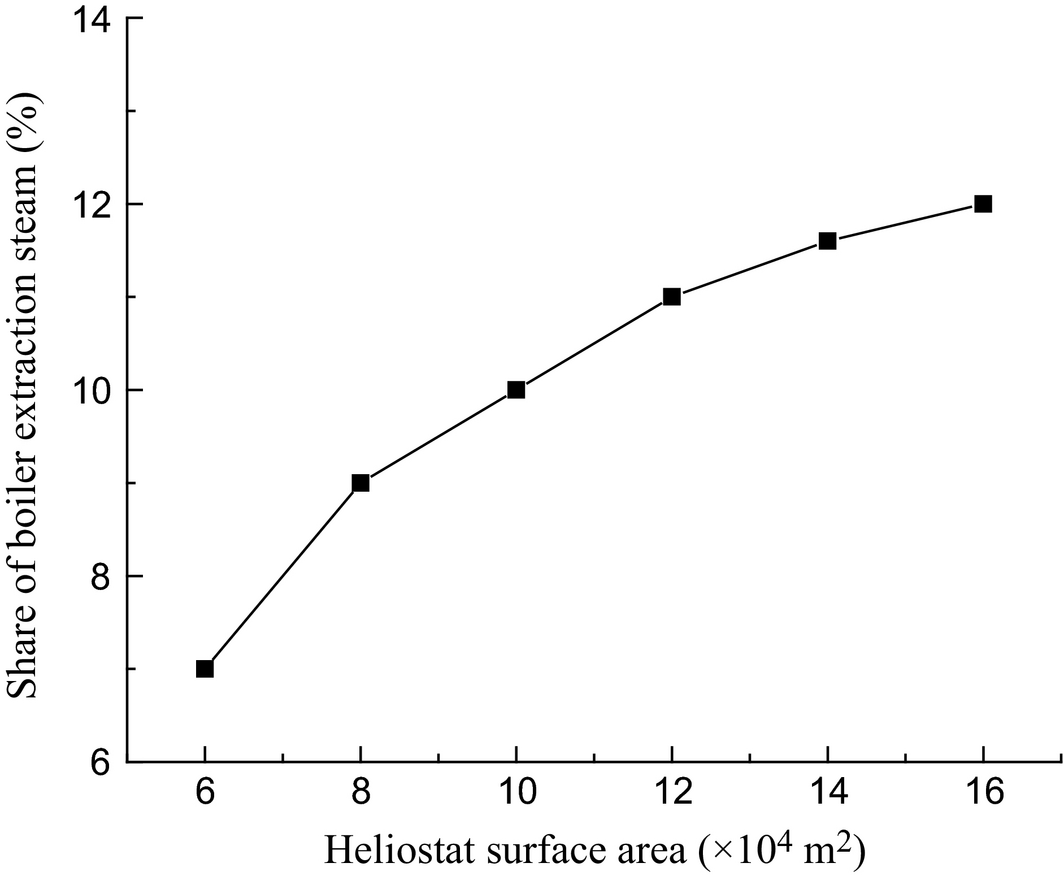Figure 13. Variation in the boiler extraction steam fraction with the heliostat surface area.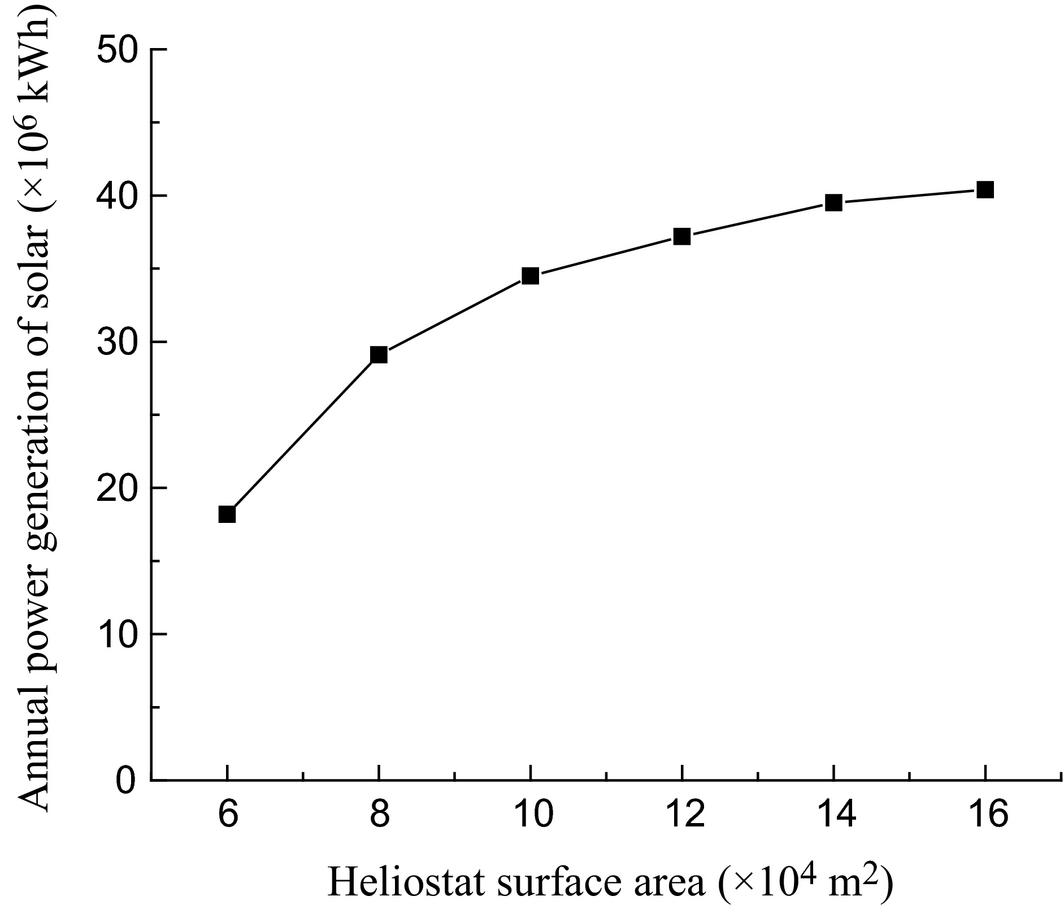Figure 14. Variation in annual power generation of solar with the heliostat surface area.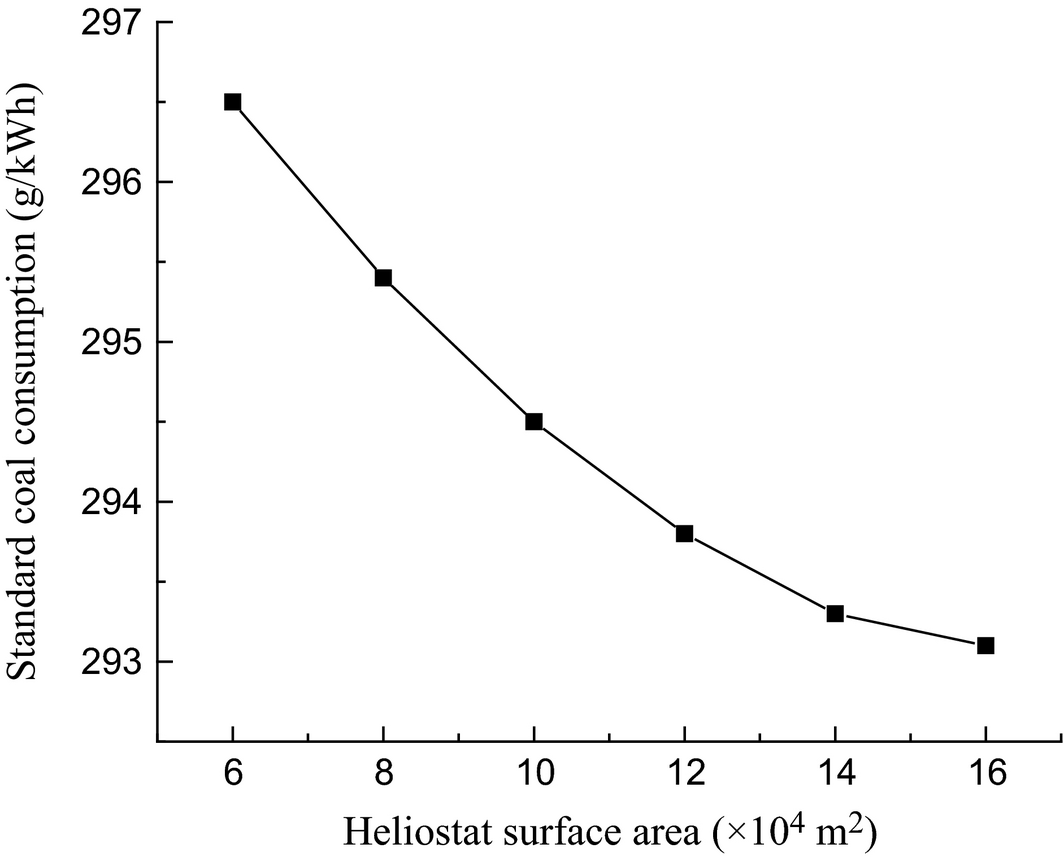Figure 15. Variation in standard coal consumption with the heliostat surface area.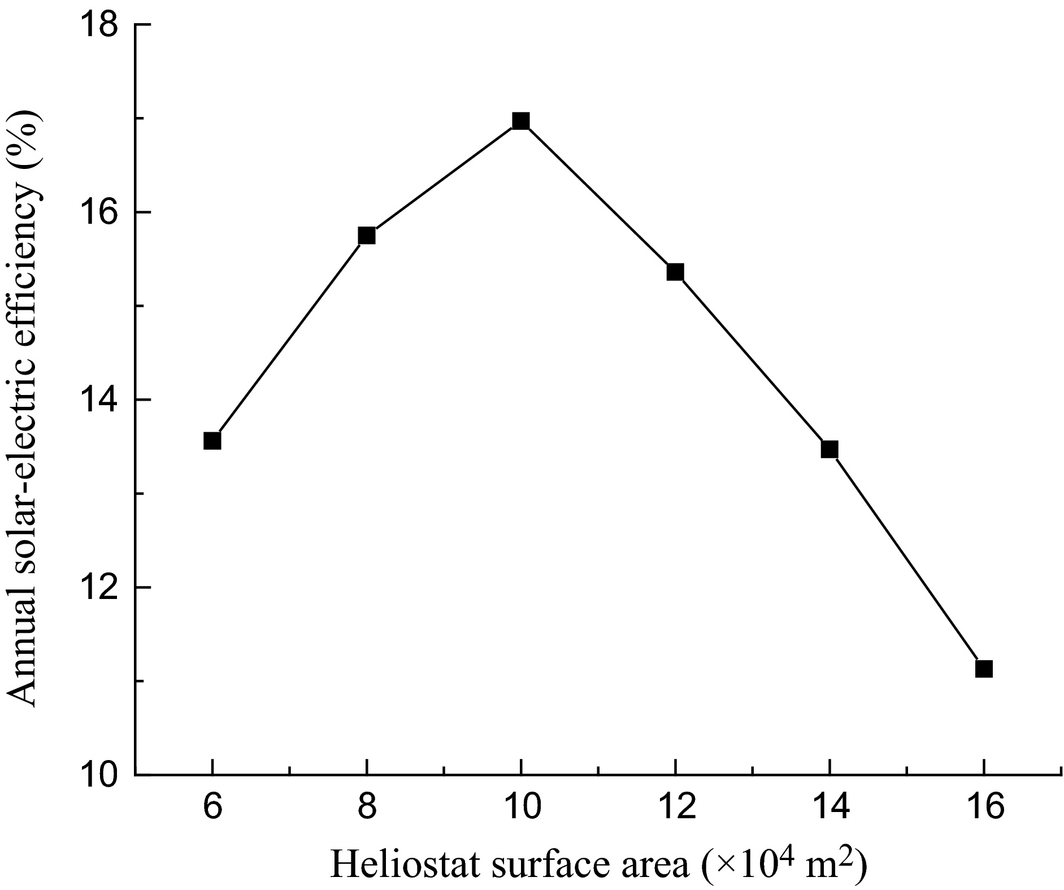Figure 16. Variation in annual solar-to-electric efficiency with the heliostat surface area.

Figure 14 shows that the annual power generation from the solar energy increases quickly at the beginning, and then the growth rate slows down with the increase in the heliostat surface area. Figure 15 shows that the standard coal consumption rate decreases quickly at first, and then the decline rate slows down with the increase in the heliostat surface area.

As shown in Figure 16, the annual solar-to-electric efficiency increases at first and then decreases with the increase in the heliostat surface area. It has a maximum value when the heliostat surface area is 100,000 m2. The reason is that as the area increases, more coal will be saved and more solar energy will be used. But if the area continues to increase, the annual solar-to-electric efficiency will decrease yet due to the increase in the unused solar energy. In other words, the growth rate of the unused solar energy is greater than the growth rate of the used solar energy. The maximum annual solar-to-electric efficiency is 16.74%. Obviously, the value of 16.74% is higher than that listed in Table 4 (15.14%) of the design condition. Moreover, the performances are shown in Table 6 under the optimal situation.

Table 6. Performance of the TSCACPG system under the optimal situation
Item Unit Value
Heliostat field area m2 101,400
Net efficiency of original plant  % 40.80
Net efficiency of solar-aided plant  % 41.77
Standard coal consumption of original unit g/kWh 301.5
Standard coal consumption of solar-aided plant g/kWh 294.5
Standard coal consumption decline g/kWh 7.0
Annual utilization hours of solar energy h 2231
Annual power generation of solar energy kWh 3.45 × 107
Annual solar-to-electric efficiency  % 16.74
Annual standard coal saved t 9655

## Conclusions

For the economic and security reasons, the TSCACPG system with the coal-saving operating mode without the energy storage is designed on the summer solstice day. The system performances of 1 day and 1 year are all carried out by using the DNI data of typical year in Dunhuang by using the simulation platform TRNSYS.

The research results show that as the heliostat area increases, the annual power generation from the solar energy increases, while the standard coal consumption rate declines. In addition, there is a maximum value of the annual solar-to-electric efficiency. In consideration of the economic costs, the heliostat field area with the maximum annual solar-to-electric efficiency is selected as the optimal value. The results show that, for the case studied, the optimal heliostat surface area is 100,000 m2, and the maximum annual solar-to-electric efficiency is 16.97%. And under the optimal situation, the standard coal consumption rate is reduced from 301.5 to 294.5 g/kWh when the solar power tower aids the power plant. This article aimed to provide the integration method and ideas for engineering projects of the tower solar collector-aided coal-fired power generation system. The achievement obtained from this paper can guide the choice of solar island scale in a specific project and provide a method of further reducing coal consumption of coal-fired power plants.

## Acknowledgments

This study has been supported by the National Nature Science Foundation Project (No. 51576062 and No. 51276063) and the National Basic Research Program of China (No. 2015CB251505).

## Nomenclature

Afield

total heliostat surface area, m2;

Ap

receiver aperture area, m2;

Apipe

surface area of piping, m2;

b

standard coal consumption rate of power supply, g/kWh;

Back

back platen superheater;

csalt

specific heat capacity of molten salt, kJ/kg. °C;

Div

division wall;

E

electrical energy output of the plant, kWh;

Eco

economizer;

epsabs

emissivity of absorber;

epspipe

emissivity of piping;

etaopt

optical efficiency;

fcooling_loss

receiver cooling loss factor;

hi,ms1

enthalpy of molten salt for #1 solar heater, kJ/kg;

ho,ms1

outlet enthalpy of molten salt for #1 solar heater, kJ/kg;

hi,ms2

inlet enthalpy of molten salt for #2 solar heater, kJ/kg;

h0,ms2

outlet enthalpy of molten salt for #2 solar heater, kJ/kg;

hi,es

inlet enthalpy of extraction steam, kJ/kg;

ho,es

outlet enthalpy of extraction steam, kJ/kg;

hi,rs

inlet enthalpy of reheat steam, kJ/kg;

ho,rs

outlet enthalpy of reheat steam, kJ/kg;

HRh

high-temperature reheater;

HSh

high-temperature superheater;

I

direct normal irradiance, kJ/h.m2;

kpipe

convective loss coefficient of piping, kJ/h.m2.K;

LRh

Low-temperature reheater;

LSh

Low-temperature superheater;

${\textstyle {\dot {m}}_{es}}$

mass flow rate of extraction steam from boiler steam-water separator, kg/h;

${\textstyle {\dot {m}}_{ms1}}$

mass flow rate of molten salt for #1 solar heater, kg/h;

${\textstyle {\dot {m}}_{ms2}}$

mass flow rate of molten salt for #2 solar heater, kg/h;

${\textstyle {\dot {m}}_{rs}}$

mass flow rate of reheat steam, kg/h;

${\textstyle {\dot {m}}_{salt}}$

total mass flow rate of molten salt, kg/h;

msc

coal consumption converted to the standard coal, kg;

PS

solar power output, W;

PZ

total output of the TSCACPG, W;

${\textstyle {\dot {Q}}_{abs}}$

solar radiation absorbed by the receiver, kJ/h;

${\textstyle {\dot {Q}}_{b}}$

heat load of the boiler, kJ/h;

${\textstyle {\dot {Q}}_{{loss}_{-}cool}}$

receiver cooling losses, kJ/h;

${\textstyle {\dot {Q}}_{{loss}_{-}pipe}}$

piping losses, kJ/h;

${\textstyle {\dot {Q}}_{{loss}_{-}rad}}$

${\textstyle {\dot {Q}}_{net}}$

thermal output of the receiver, kJ/h;

${\textstyle {\dot {Q}}_{rec}}$

the power to the receiver, kJ/h;

${\textstyle {\dot {Q}}_{S}}$

total solar projection energy, kJ/h;

${\textstyle {\dot {Q}}_{th}}$

thermal power of solar power tower, kJ/h;

${\textstyle {\overline {T}}_{abs}}$

average temperature of absorber, °C;

tamb

ambient temperature, °C;

tin

inlet temperature of molten salt for receiver, °C;

tout

outlet temperature of molten salt for receiver, °C;

WW

water wall;

## Greek symbols

Γ

a control parameter describing the fraction of the field in track;

σ

Stefan constant, σ  =  5.67 × 10−8W/(m2.K4);

ρfield

heliostat field reflectivity;

ηfield

the heliostat field efficiency;

ηrec

ηref

the thermal efficiency of the original coal-fired power unit;

ηse

the solar-to-electric efficiency;

## Conflict of Interest

None declared.

### Document informationPublished on 01/06/17
Submitted on 01/06/17

Licence: Other

### Document Score0

Views 10
Recommendations 0